×
Get Full Access to Fluid Mechanics - 2 Edition - Chapter 3 - Problem 19p
Get Full Access to Fluid Mechanics - 2 Edition - Chapter 3 - Problem 19p

×

# The basic barometer can be used to measure the height of aISBN: 9780071284219 39

## Solution for problem 19P Chapter 3

Fluid Mechanics | 2nd Edition

• Textbook Solutions
• 2901 Step-by-step solutions solved by professors and subject experts
• Get 24/7 help from StudySoup virtual teaching assistantsFluid Mechanics | 2nd Edition

4 5 1 239 Reviews
29
5
Problem 19P

The basic barometer can be used to measure the height of a building. If the barometric readings at the top and at the bottom of a building are 730 and 755 mmHg, respectively, determine the height of the building. Assume an average air density of 1.18 $$\mathrm{kg} / \mathrm{m}^{3}$$.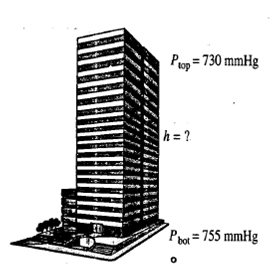Step-by-Step Solution:

Step 1 of 4

The barometer is a  scientific  device which is used to find out the pressure at a point.  The barometer   reading   at the top of the building is 730 mmHg and at the  bottom is  755 mmHg.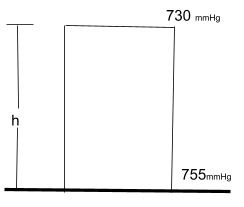The atmospheric pressure at the top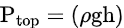The density of mercury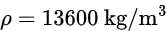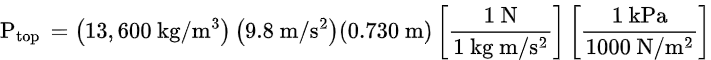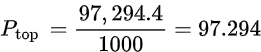Step 2 of 4

Step 3 of 4

## Discover and learn what students are asking

Calculus: Early Transcendental Functions : Antiderivatives and Indefinite Integration
?In Exercises 7–10, complete the table to find the indefinite integral. Original Integral Rewrite I

Statistics: Informed Decisions Using Data : Estimating a Population Proportion
?A ____________ is the value of a statistic that estimates the value of a parameter.

Statistics: Informed Decisions Using Data : Inference about the Difference between Two Medians: Dependent Samples
?Effect of Aspirin on Blood Clotting Blood clotting is due to a sequence of chemical reactions. The protein thrombin initiates blood clotting by workin

Unlock Textbook Solution

Enter your email below to unlock your verified solution to:

The basic barometer can be used to measure the height of a